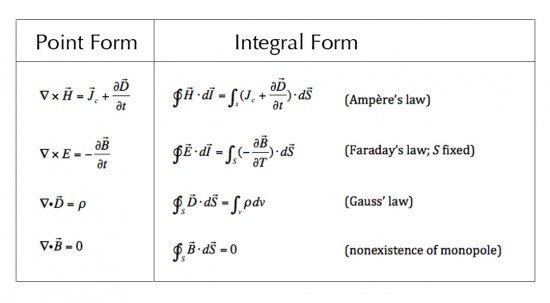## Appendix II: The Electro-magnetic Field EquationsIntroduction Maxwell’s Equations and the Lorentz Force Law together comprise the e/m field equations; i.e., those equations determining the interactions of charged particles in the vicinity of electric and magnetic fields and the resultant effect of those interactions on the values of the e/m field. For ease of explanation, the…